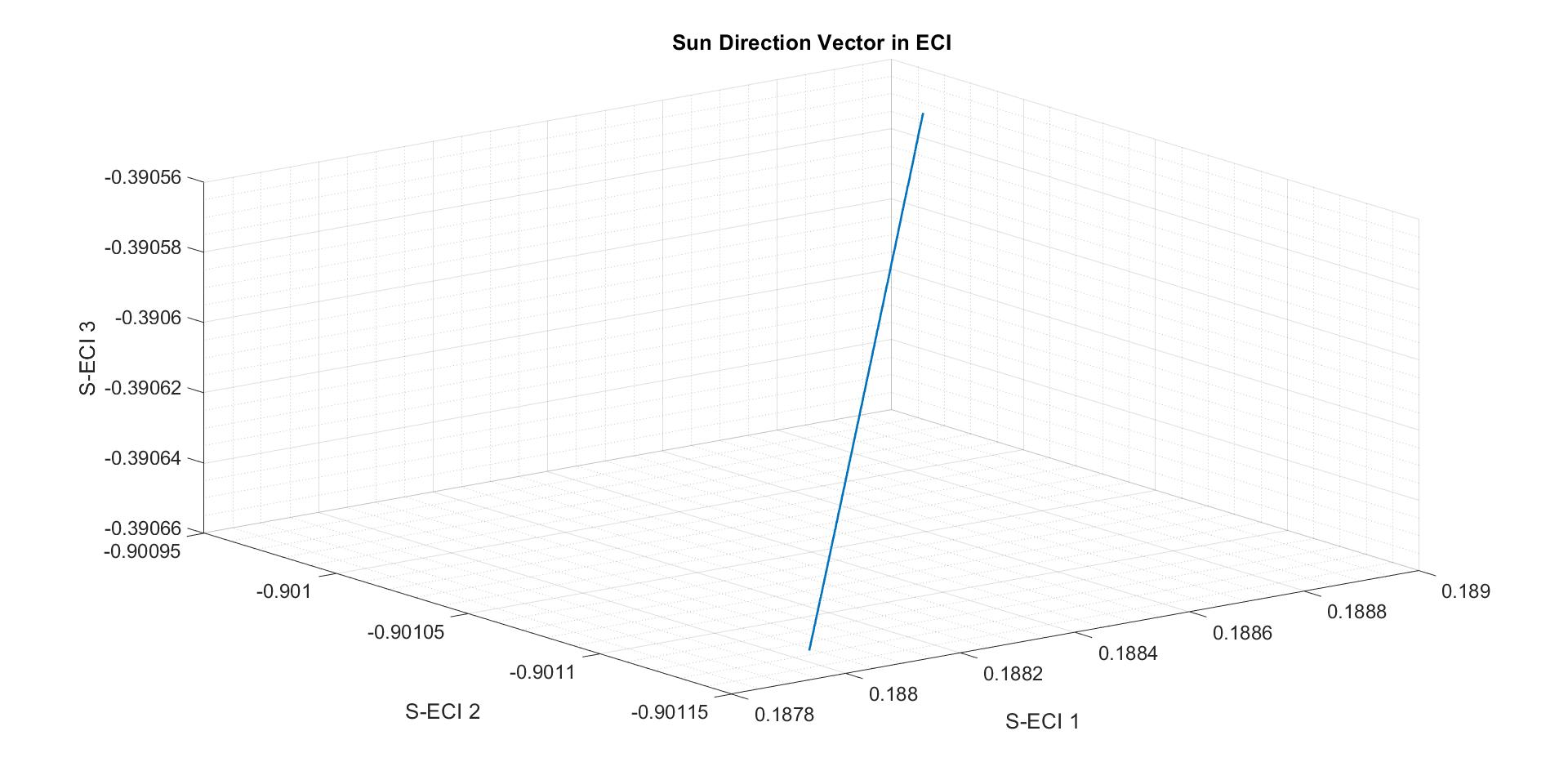## Investigation of the Sun Direction Vector in ECI

Version 1.0.0 (1.64 KB) by
The sun model calculates the sun line in the inertial reference system and determines whether the sun is obstructed by the Earth at any give

Updated 7 Jul 2022

• The sun model calculates the sun line in the inertial reference system and determines whether the sun is obstructed by the Earth at any given time into the mission. Due to the spacecraft orbiting the Earth and the Earth itself revolving about the sun, the inertial sun vector changes minutely while the satellite travels along its orbit. However, for LEO satellites with negligible orbit radius compared to the astronomical unit these cyclic changes, in the order of few arcseconds, are well below the accuracy of the sun sensors and the required accuracy of the reference model; this enables the useful simplification of the sun model by assuming the sun line to be independent of the position of the spacecraft and hence always parallel to the vector from the Earth to the sun. The following standard algorithm describes the true motion of the Earth around the inertially fixed sun in the form of the sun orbiting the Earth being fixed in inertial space. The right ascension of the ascending node of this virtual orbit of the sun around the Earth is 0° by definition. The reference epoch of this first-order model is the 1st of January 2000, 12:00:00 pm, or 2451545.0 Julian Date (JD).### Cite As

Anil Taha Balkir (2023). Investigation of the Sun Direction Vector in ECI (https://www.mathworks.com/matlabcentral/fileexchange/114710-investigation-of-the-sun-direction-vector-in-eci), MATLAB Central File Exchange. Retrieved .

##### MATLAB Release Compatibility
Created with R2020a
Compatible with any release
##### Platform Compatibility
Windows macOS Linux